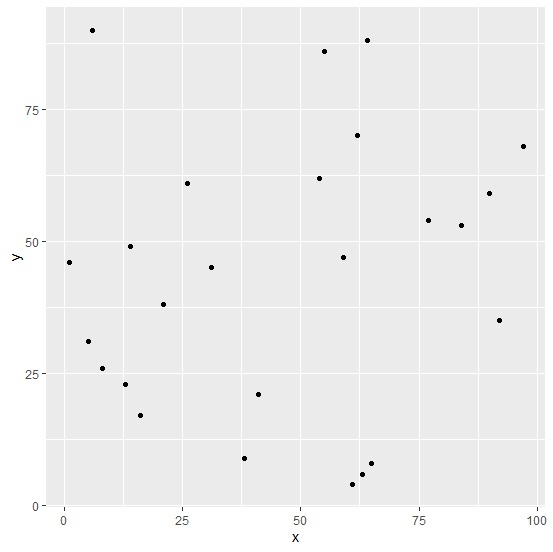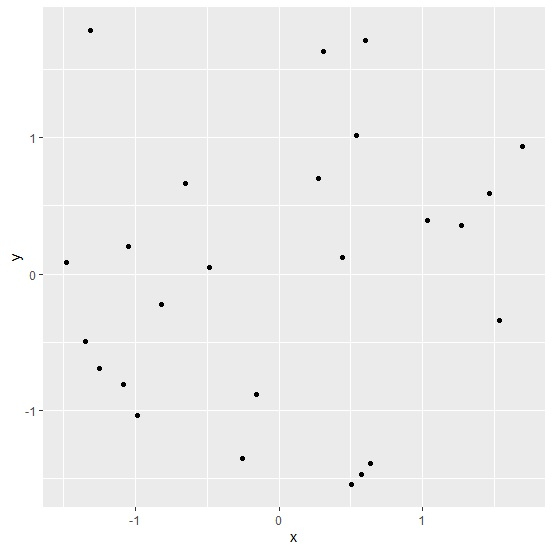# How to create scatterplot by standardizing the columns of a data frame using ggplot2 R?

To create scatterplot by standardizing the columns of a data frame using ggplot2 R, we can follow the below steps −

• First of all, create a data frame.

• Then, create the scatterplot using ggplot2 with raw values.

• After that, create the scatterplot with scale function.

## Create the data frame

Let’s create a data frame as shown below −

Live Demo

x<-sample(1:100,25)
y<-sample(1:100,25)
df<-data.frame(x,y)
df

On executing, the above script generates the below output(this output will vary on your system due to randomization) −

## Output

   x  y
1  61 4
2  26 61
3  5  31
4  1  46
5  31 45
6  92 35
7  64 88
8  63 6
9  90 59
10 54 62
11 55 86
12 16 17
13 97 68
14 62 70
15 8 26
16 38 9
17 65 8
18 21 38
19 77 54
20 6 90
21 13 23
22 59 47
23 14 49
24 41 21
25 84 53

## Create the scatterplot

Using geom_point function of ggplot2 package to create the scatterplot between x and y −

x<-sample(1:100,25)
y<-sample(1:100,25)
df<-data.frame(x,y)
library(ggplot2)
ggplot(df,aes(x,y))+geom_point()

## Output## Create the scatterplot after standardizing the columns

Using scale function inside aes of geom_point to create the scatterplot between standardized values of x and y −

x<-sample(1:100,25)
y<-sample(1:100,25)
df<-data.frame(x,y)
library(ggplot2)
ggplot(df,aes(x,y))+geom_point(aes(scale(x),scale(y)))

## Output